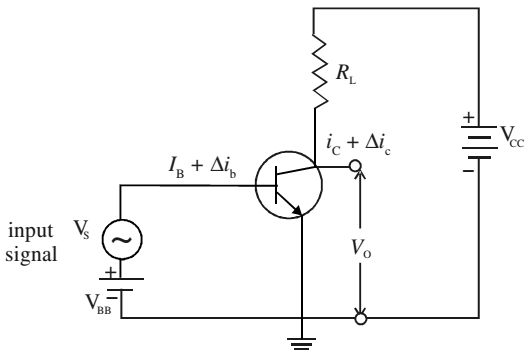# Transistor as an Amplifier

An electrical signal is voltage or current, which is coded with some useful information. For example, when you speak in front of a microphone, its diaphragm vibrates and induces a very small voltage in its coil, depending on the intensity of sound. This induced voltage appears as a weak signal and can not operate a loudspeaker to reproduce sound. To make it intelligible, it is fed into a device called amplifier. The amplifier increases the level of input signal and gives out magnified output.

If Vi is the input signal voltage fed to the amplifier and VO denotes the amplified output, their ratio is called voltage gain.

AV = VO/Vi

Similarly, the current gain and power gain is defined as

AI = IO/Ii

Ap = PO/Pi

An n-p-n transistor is used in CE mode. Its collector is reverse biased through the load resistance RL by the battery VCE. When a base current IB flows, some collector current IC will start flowing. On decreasing IB, a stage will be reached when IC becomes almost zero. This is the lower limit of variation of IB. Similarly, on increasing IB again, a stage of saturation is reached and IC stops increasing. This corresponds to the upper limit of variation of IB.For faithful amplification of input signal, a base current equal to the mean of these two limiting values of IB is passed through the base by forward biasing it with battery VBB. This is called biasing of the base. A signal source providing an input signal Vs connected in series with VBB.

Due to addition of oscillating signal voltage Vs to VBB, the base current changes by an amount ∆ib around the dc biasing current IB. The signal voltage is kept low so that the signal current ∆ib if added and subtracted from IB does not cross the upper and lower limits of the base current variation. Otherwise, the transistor will go into cut off or saturation region and the amplified output will be highly distorted and noisy.

∆ib = Vs/ri

where ri is the input impedance. This change in base current ∆ib results in a large change in collector current, say ∆ic given by

∆ic = β∆ib = βVs/ri

where β is the ac current amplification factor, equal to ∆ic/∆ib.

Vs = ∆ic × ri

By applying Kirchoff’s law to the output circuit,

VCC = VCE + ICRL

On differentiating,

dVCC= dVCE + dIC × RL

Since VCC is constant, dVCC = 0. Therefore,

dVCE = –dIC × RL

But dVCE is the change in output ∆V0 and dIC in ic. Therefore,

∆Vo = – ∆ic × RL

The voltage gain AV of the amplifier is given by

AV = –β × RL/ri

The ratio β/ri is called transconductance of transistor and is denoted by gm.

AV = – gm × RL

The negative sign indicates that input and output are in opposite phase, i.e. they differ in phase by 180°. The power gain is given by

AP = AI × AV = β × AV

Power gain does not mean that the law of conservation of energy is violated in an amplifier. The ac power output of the amplifier is more than the ac input signal power but this gain is achieved at the cost of dc power supplied by the voltage source.